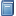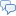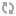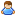BuchempfehlungMikrocomputertechnik mit Controllern der Atmel AVR-RISC-Familie
Umfassend, aber leicht verständlich führt dieses Buch in die Programmierung von ATMEL AVR Mikrocontrollern ein. [Mehr Infos...]FreeBASIC-Chat
Es sindBenutzer im FreeBASIC-Chat online.
(Stand:  )

# fb:porticula NoPaste

## plotter_mod.bi

 Uploader:grindstone Datum/Zeit: 01.05.2015 11:02:28

```/'   Copyright (c) 2014 by nemored   Permission is hereby granted, free of charge, to any person obtaining a copy of this software and associated documentation files (the "Software"), to deal in the Software without restriction, including without limitation the rights to use, copy, modify, merge, publish, distribute, sublicense, and/or sell copies of the Software, and to permit persons to whom the Software is furnished to do so, subject to the following conditions:   The above copyright notice and this permission notice shall be included in all copies or substantial portions of the Software.   THE SOFTWARE IS PROVIDED "AS IS", WITHOUT WARRANTY OF ANY KIND, EXPRESS OR IMPLIED, INCLUDING BUT NOT LIMITED TO THE WARRANTIES OF MERCHANTABILITY, FITNESS FOR A PARTICULAR PURPOSE AND NONINFRINGEMENT. IN NO EVENT SHALL THE AUTHORS OR COPYRIGHT HOLDERS BE LIABLE FOR ANY CLAIM, DAMAGES OR OTHER LIABILITY, WHETHER IN AN ACTION OF CONTRACT, TORT OR OTHERWISE, ARISING FROM, OUT OF OR IN CONNECTION WITH THE SOFTWARE OR THE USE OR OTHER DEALINGS IN THE SOFTWARE.      Version 2014-08-05      ---      The capabilities to quote the colour of the graph and to draw it without a coordinate cross      were added by grindstone in April 2015 '/ #include once "calculate.bi" #include once "fbgfx.bi" #ifndef PLOTTER_COLOR_AXIS   #define PLOTTER_COLOR_AXIS rgb(127, 127, 127) #endif #ifndef PLOTTER_COLOR_GRAPH   #define PLOTTER_COLOR_GRAPH rgb(255, 255, 255) #endif #ifndef PLOTTER_COLOR_BACKGROUND   #define PLOTTER_COLOR_BACKGROUND rgb(0, 0, 0) #endif declare function plotFunction overload(term as string, xStart as double, xEnd as double, yStart as double, yEnd as double, _                  xWidth as integer, yWidth as integer, xStep as double = 1, _yStep as double = 1, _                  gcolor As UInteger = PLOTTER_COLOR_GRAPH, nocc As Integer = 0) as FB.Image ptr declare function plotFunction(img as FB.Image ptr, term as string, xStart as double, xEnd as double, _                  yStart as double, yEnd as double, xStep as double = 1, yStep as double = 1, _                  gcolor As UInteger = PLOTTER_COLOR_GRAPH, nocc As Integer = 0) as FB.Image ptr function plotFunction(term as string, xStart as double, xEnd as double, yStart as double, yEnd as double, _                       xWidth as integer, yWidth as integer, xStep as double = 1, yStep as double = 1, _                       gcolor As UInteger = PLOTTER_COLOR_GRAPH, nocc as integer = 0) as FB.Image ptr   dim as FB.image ptr img = imagecreate(xWidth, yWidth, PLOTTER_COLOR_BACKGROUND)   return plotFunction(img, term, xStart, xEnd, yStart, yEnd, xStep, yStep, gcolor, nocc) end function function plotFunction(img as FB.Image ptr, term as string, xStart as double, xEnd as double, _                       yStart as double, yEnd as double, xStep as double = 1, yStep as double = 1, _                       gcolor As UInteger = PLOTTER_COLOR_GRAPH, nocc As Integer = 0) as FB.Image ptr   if xStep <= 0 or yStep <= 0 then draw string img, (1, 1), "ERROR", PLOTTER_COLOR_GRAPH : return img   ' read image proportions   dim as integer xWidth, yWidth   if img = 0 then screeninfo xWidth, yWidth else imageinfo img, xWidth, yWidth   ' coordinate system   dim as single xFactor = xWidth / (xEnd - xStart)   dim as single yFactor = yWidth / (yEnd - yStart)   dim as integer xAxis = yWidth + yWidth * yStart / (yEnd - yStart) - 1   dim as integer yAxis = - xStart * xFactor     if nocc = 0 then       if xAxis >= 0 and xAxis < yWidth then         line img, (0, xAxis)-(xWidth-1, xAxis), PLOTTER_COLOR_AXIS         line img, -step(-5, -5), PLOTTER_COLOR_AXIS         line img, step(5, 5)-step(-5, 5), PLOTTER_COLOR_AXIS         for i as integer = int(xStart) to int(xEnd)+1 step xStep           line img, ((i-xStart)*xFactor, xAxis-2)-step(0, 4), PLOTTER_COLOR_AXIS         next       end if       if yAxis >= 0 and yAxis < xWidth then         line img, (yAxis, yWidth-1)-(yAxis, 0), PLOTTER_COLOR_AXIS         line img, -step(-5, 5), PLOTTER_COLOR_AXIS         line img, step(5, -5)-step(5, 5), PLOTTER_COLOR_AXIS         for i as integer = int(yStart) to int(yEnd)+1 step yStep           line img, (yAxis-2, (i-yStart)*yFactor)-step(4, 0), PLOTTER_COLOR_AXIS         next       end If     endif   ' graph   dim as double value, valueOld   valueOld = yEnd + 1   for i as integer = 0 to xWidth     value = Calculate.eval("x=" & (i-yAxis)/xFactor & ";" & term)     if Calculate.CalcError > 0 then       valueOld = yEnd + 1     else       if value > yStart and value <= yEnd then         if valueOld > yStart and valueOld <= yEnd then           'line img, -(i, xAxis - value*yFactor), PLOTTER_COLOR_GRAPH           line img, -(i, xAxis - value*yFactor), gcolor         else           'pset img, (i, xAxis - value*yFactor), PLOTTER_COLOR_GRAPH           pset img, (i, xAxis - value*yFactor), gcolor         end if         valueOld = value       end if     end if   next   if Calculate.CalcError > 0 and Calculate.CalcError <> Calculate.ErrorIllegalValue then     draw string img, (1, 1), "ERROR", PLOTTER_COLOR_GRAPH   end if   return img end function```